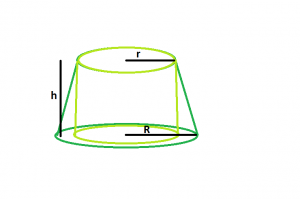# Largest right circular cylinder within a frustum

Given a frustum of height, top-radius& base-radius. The task is to find the volume of biggest right circular cylinder that can be inscribed within it.

Examples:

```Input  : r = 5, R = 10, h = 4
Output : 314

Input : r = 7, R = 11, h = 6
Output : 923.16
```

## Recommended: Please try your approach on {IDE} first, before moving on to the solution.Approach:
Let:

• The height of the cylinder = h1
• Radius of the cylinder = r1

From the figure it is clear that:

• Height of the cylinder = Height of frustum

So,

```h1 = h
r1 = r```

Below is the implementation of the above approach:

## C++

 `// C++ Program to find the biggest right circular cylinder ` `// that can be fit within a frustum ` `#include ` `using` `namespace` `std; ` ` `  `// Function to find the biggest right circular cylinder ` `float` `cyl(``float` `r, ``float` `R, ``float` `h) ` `{ ` `    ``// radii and height cannot be negative ` `    ``if` `(h < 0 && r < 0 && R < 0) ` `        ``return` `-1; ` ` `  `    ``// radius of right circular cylinder ` `    ``float` `r1 = r; ` `    ``// height of right circular cylinder ` `    ``float` `h1 = h; ` `    ``// volume of right circular cylinder ` `    ``float` `V = 3.14 * ``pow``(r1, 2) * h1; ` ` `  `    ``return` `V; ` `} ` ` `  `// Driver code ` `int` `main() ` `{ ` `    ``float` `r = 7, R = 11, h = 6; ` ` `  `    ``cout << cyl(r, R, h) << endl; ` ` `  `    ``return` `0; ` `} `

## Java

 `// Java Program to find the biggest right circular cylinder ` `// that can be fit within a frustum ` ` `  `import` `java.io.*; ` ` `  `class` `GFG { ` ` `  ` `  `// Function to find the biggest right circular cylinder ` ` ``static` `float` `cyl(``float` `r, ``float` `R, ``float` `h) ` `{ ` `    ``// radii and height cannot be negative ` `    ``if` `(h < ``0` `&& r < ``0` `&& R < ``0``) ` `        ``return` `-``1``; ` ` `  `    ``// radius of right circular cylinder ` `    ``float` `r1 = r; ` `    ``// height of right circular cylinder ` `    ``float` `h1 = h; ` `    ``// volume of right circular cylinder ` `    ``float` `V = (``float``)(``3.14` `* Math.pow(r1, ``2``) * h1); ` ` `  `    ``return` `V; ` `} ` ` `  `// Driver code ` `    ``public` `static` `void` `main (String[] args) { ` `            ``float` `r = ``7``, R = ``11``, h = ``6``; ` ` `  `    ``System.out.print( cyl(r, R, h)); ` `    ``} ` `} ` `// This code is contributed by anuj_67.. `

## Python3

 `# Python3 Program to find the biggest right circular cylinder ` `# that can be fit within a frustum ` ` `  `# Function to find the biggest right circular cylinder ` `def` `cyl(r, R, h) : ` ` `  `    ``# radii and height cannot be negative ` `    ``if` `(h < ``0` `and` `r < ``0` `and` `R < ``0``) : ` `        ``return` `-``1` ` `  `    ``# radius of right circular cylinder ` `    ``r1 ``=` `r ` `    ``# height of right circular cylinder ` `    ``h1 ``=` `h ` `    ``# volume of right circular cylinder ` `    ``V ``=` `3.14` `*` `pow``(r1, ``2``) ``*` `h1 ` ` `  `    ``return` `round``(V,``2``) ` ` `  ` `  `# Driver code ` `if` `__name__ ``=``=` `"__main__"` `: ` ` `  `    ``r, R, h ``=` `7``, ``11``, ``6` ` `  `    ``print``(cyl(r, R, h)) ` ` `  `# This code is contributed by Ryuga `

## C#

 `// C# Program to find the biggest right circular cylinder ` `// that can be fit within a frustum ` `using` `System; ` ` `  `class` `GFG { ` ` `  ` `  `// Function to find the biggest right circular cylinder ` `static` `float` `cyl(``float` `r, ``float` `R, ``float` `h) ` `{ ` `    ``// radii and height cannot be negative ` `    ``if` `(h < 0 && r < 0 && R < 0) ` `        ``return` `-1; ` ` `  `    ``// radius of right circular cylinder ` `    ``float` `r1 = r; ` `    ``// height of right circular cylinder ` `    ``float` `h1 = h; ` `    ``// volume of right circular cylinder ` `    ``float` `V = (``float``)(3.14 * Math.Pow(r1, 2) * h1); ` ` `  `    ``return` `V; ` `} ` ` `  `// Driver code ` `    ``public` `static` `void` `Main () { ` `            ``float` `r = 7, R = 11, h = 6; ` ` `  `    ``Console.WriteLine( cyl(r, R, h)); ` `    ``} ` `} ` `// This code is contributed by anuj_67.. `

## PHP

 `

Output:

```923.16
```

My Personal Notes arrow_drop_upCheck out this Author's contributed articles.

If you like GeeksforGeeks and would like to contribute, you can also write an article using contribute.geeksforgeeks.org or mail your article to contribute@geeksforgeeks.org. See your article appearing on the GeeksforGeeks main page and help other Geeks.

Please Improve this article if you find anything incorrect by clicking on the "Improve Article" button below.

Improved By : vt_m, AnkitRai01, Code_Mech

Article Tags :
Practice Tags :

Be the First to upvote.

Please write to us at contribute@geeksforgeeks.org to report any issue with the above content.